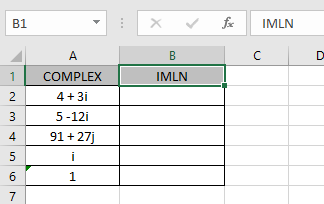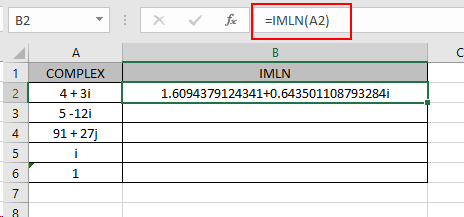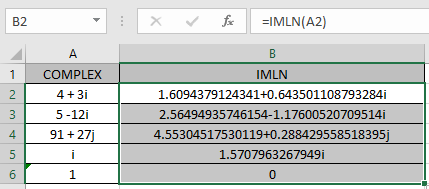# How to use the IMLN Function in Excel

COMPLEX number (inumber) in excel derived for mathematical number having real and imaginary coefficients. In mathematics we call it the coefficient of i or j (iota).
i = (-1)1/2
Square root of negative number is not possible, so for calculation purpose, ?-1 is named as imaginary and call it iota (i or j). For calculation of some term like shown below.

A = 2 + (-25)1/2

A = 2 + (-1 * 25)1/2

A = 2 + (-1 * 5 * 5)1/2

A = 2 + 5 * (-1)1/2

X + iY = 2 + 5i

This here equation is a Complex number (inumber) having 2 different parts called real part imaginary part

The coefficient of iota (i) which is 5 is called as imaginary part and the other part 2 is called the real part of the complex number.
Complex number (inumber) is written in the X + iY format.

Natural logarithm of a complex number (X + iY) is given by

loge ( X +iY) = loge(X2 +Y2)1/2+ i tan-1(Y/X)

Here X & Y are the coefficients of the real & imaginary part of the complex number (inumber).

Here:

1. log at the base e is called the natural logarithm of a number where value of e =  2.7182..(approx).
2. Coefficient of iota is inverse tan function of ( Y / X ) is tan-1(Y/X)which returns the angle in radians.

ln ( X +iY) = ln (X2 +Y2)1/2 + i tan-1(Y/X)
The IMLN function returns the complex natural logarithm of the complex number (inumber) having both real & imaginary part.

Syntax:

=IMLN (inumber)

inumber : complex number for which you want the complex natural logarithm.

Let’s understand this function using it in an example.Here we have values where we need to get the complex natural logarithm of the input complex number (inumber)
Use the formula:

=IMLN (A2)

A2 : complex number (inumber) provided as cell reference.As you can see the complex number having real_num = 4 & imaginary part = 3. The formula returns the complex natural logarithm of the complex number.
natural logarithm of a complex number (4 + 3i) =
loge (4 + 3i) = ln (4 +3i) = ln (42 +32)1/2 + i tan-1( 3 / 4 )
Now copy the formula to the other remaining cells using Ctrl + D shortcut key.As you can see the IMLN function formula giving results just fine.

The table here explains more about the input real & imaginary part

 inumber Real part (X) Imaginary part (Y) i = 0 + 1i 0 1 1 = 1 + 0i 1 0

Note:
The formula returns the #NUM! error if the complex number doesn’t have lower case i or j (iota) otherwise the excel treats it as text rather than a complex number.
Hope you understood how to use IMLN function and referring cell in Excel. Explore more articles on Excel mathematical functions here. Please feel free to state your query or feedback for the above article.

Related Articles

Excel LN function

How to use the IMEXP Function in Excel

How to use the IMCONJUGATE Function in Excel

How to use the IMARGUMENT Function in Excel

Popular Articles

Edit a dropdown list

If with conditional formatting

If with wildcards

Vlookup by date

Terms and Conditions of use

The applications/code on this site are distributed as is and without warranties or liability. In no event shall the owner of the copyrights, or the authors of the applications/code be liable for any loss of profit, any problems or any damage resulting from the use or evaluation of the applications/code.